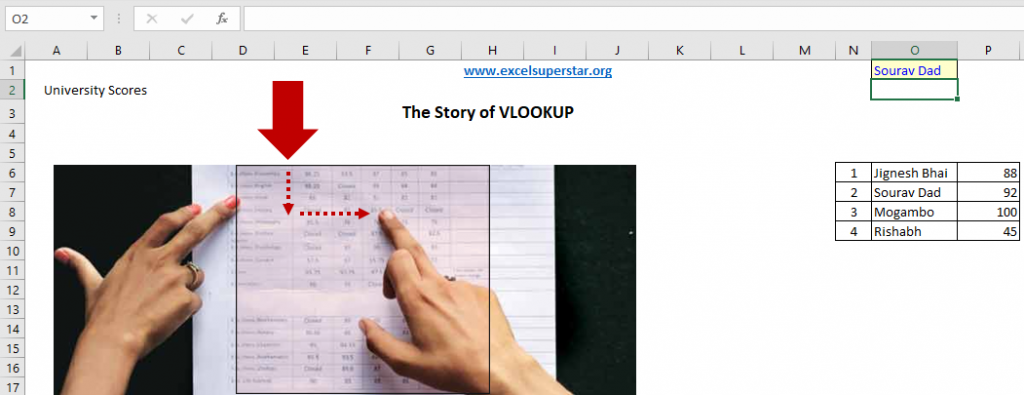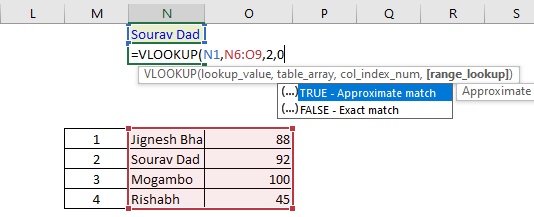# How to use VLOOKUP Formula in ExcelThere are many people out there who have learned VLOOKUP but they are getting confused when and where to apply it in Excel. So to get rid of your confusion I will be talking about VLOOKUP Step 1 which is absolute basics and also I will show you how to How to use this Foundation VLOOKUP in Excel

How to use VLOOKUP in Excel?MS Excel Course in Hindi

• Get 2-Year Access with unlimited views of 220+ videos
• 100+ case studies you won't find ANYWHERE

To use VLOOKUP in Excel. I will be going to explain you through my type of story by which you will understand when and where to apply it in Excel

So here I have 4 students in my story

 1 Jignesh Bhai 88 2 Sourav Dada 92 3 Mogambo 100 4 Rishabh 45

Step 1: Copy the name of Sourav Dada
Step 2: Then press Ctrl + F
Step 3: A pop up will open paste the name on it
Step 4: Click on Find Next
Step 5: Lastly, the cursor will navigate and click on your name in order to know the answer

I think that the name should come automatically which means if I change my name from Sourav Dada to Jignesh Bhai then I should get my answer.

So to quickly find your answer, first let us go through a detailed process to apply VLOOKUP and find your answer in Excel

How to apply the VLOOKUP formula to find an answer in Excel?

To apply VLOOKUP Formula in Excel what I have done is:

Step 1: Firstly I have written this VLOOKUP FormulaNow the first parameter is saying lookup value and not lookup values that mean 1 time only 1 value or 1 cell so what we will do is

Step 2 – Next I have chosen the cell to find an answer so it becomes =VLOOKUP[N1,

Now VLOOKUP tells us that whichever LOOKUP value I have chosen that LOOKUP value must be there in the first part of the column. If you have selected the entire column then you will not find your answer

Step 3 – So I have selected column 1 and column 2 which becomes =VLOOKUP[N1,N6:09,

Now the formula link is becoming like that =VLOOKUP[N1,N6:09, which means LOOKUP value is Sourav Dada and the name should be there in this table. If the name is there in 1 column then we have to find the answer from the 2 columns so what I will write is

Step 4 – I will write the above formula and add the value 2 along with comma which becomes =VLOOKUP[N1,N6:09,2,

Now after writing the above formula now the range LOOKUP is saying True and False in which

TRUE= Appropriate Match
FALSE = Exact Match

Step 5- So here I will select FALSE because my focus is one exact match which becomes =VLOOKUP[N1,N6:09,2, FALSE]So by following the above-given step by step process using VLOOKUP in Excel you will get your value as an answer easily without any trouble

Conclusion:

So that’s it by following the step by step procedure in our blog you will understand How to use VLOOKUP in Excel and now you can do it easily on your own without any hesitation. So start practicing now and if you have any doubt then feel free to comment us I will be glad to help you out.

5% Discount
No prize
30% Discount
Almost!
10% Discount
Almost
70% Discount
No luck today
Almost!
50% Discount
No prize
Unlucky
Get your chance to win a price! on Excel Mastery Program Bundle